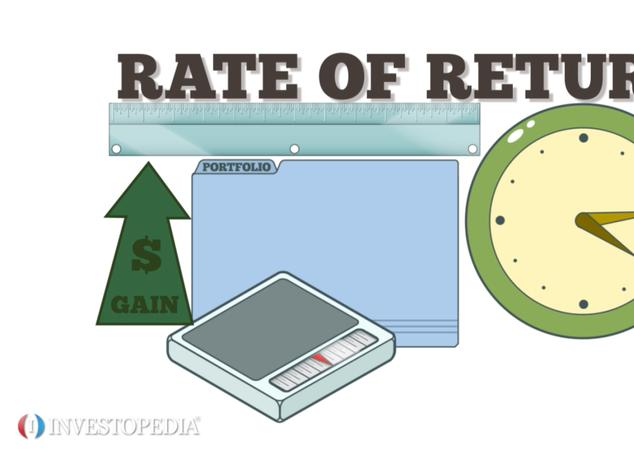Home Technology Average return time

# Average return time

Mean recurrent time (mean recurrent time) The expected value of the time that a Markov chain returns to a given state i after it departs. Let i be a recurrent state, then {only, n=1, 2 , 3, w) gives the probability distribution of the time for the Markov chain to return to i for the first time after starting from state i. The mathematical expectation of this distribution is the average return time of state 7 (see "Probability of first passing "And "average first elapsed time").